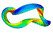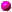## Theory of Partial Differential EquationsVarious regimes of soliton propagation in optical fibers and gratings are modeled numerically by scalar, vector, and periodic modules of the nonlinear Schrodinger (NLS) equation. Linearization of the NLS equations results in a matrix eigenvalue problem which typically has the unstable eigenvalues leading to exponential growth of small perturbations. Potential commercial use of solitons in optical fibers, wave couplers, photonic devices and semiconductor lasers can be achieved in the regime where the soliton propagation is observed to be stable.

The challenging problem to detect soliton instabilities in different nonlinear optical structures was attacked simultaneously by many research groups during the last decade. Up to the date, basic properties of the soliton spectrum in the NLS equations have been understood and classified. Many asymptotic and numerical approaches were developed to explain different mechanisms for instabilities, bifurcations, resonances, radiative decays and oscillatory nonlinear dynamics of soliton signals.

It is now the time to complete the most general theory of soliton instabilities and to implement the basic principles and methods into powerful software packages. The software is to be directed for solving new modules of the NLS equations that arise in design of new effective optical devices in fiber and photonic optics communications.Linearization AnalysisEnergy Analysis